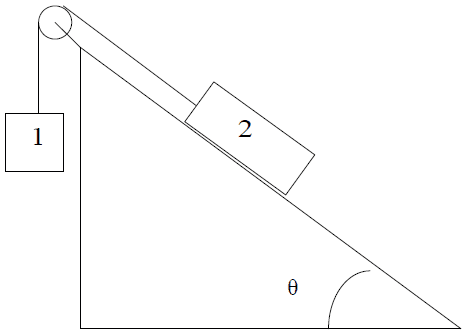# Tension of a string with a pulley

## Homework Statement

Two masses are connected by a string over a pulley as shown below. The incline is
frictionless and makes a 50 degree angle from the horizontal. If m1 = 3.00 kg and m2 = 8.00 kg, find the tension in the string.F = ma

## The Attempt at a Solution

I thought the tension in the string would be the same as the weight of m1, which is 29.4N, but that is not the correct answer. I am not sure what to try from here.

m1 accelerates, so you need to take that into consideration.
First write up the equations of motion of the two blocks.
i.e. m1*a=...
and m2*a=...

I got that that a equals 2.79m/s², so:

m1*a = 3 * 2.79 = 8.37N
m2*a = 8 * 2.79 = 22.32N

Is that what you mean? Is that right?

Yes, and now you can express the tension of the string. m1*a=m1*g-T

That got me the correct answer! Thanks so much for your help!

Last edited: